# Momentum indicator - page 53

hallo

im looking for indicator mtf momentum histogram flattrend alert change at 100 ( similar macd https://www.mql5.com/en/forum/175290/page52).

does anybody have it?

thanks for any feedback255627

In a 21:7 issue of TASC, Chris Satchwell in his article "A Generic Tool For Technical Analysis - Regularization" describes 2 indicators : Regularized EMA (which, for example, can be found for metatrader in AllAverages indicator by igorad) and Regularized momentum.

Regularization is primarily a mean of smoothing values and it seems that in the regularized momentum indicator it shows its best sides. Smoothing done that way is adding minimal lag to momentum indicator and it then becomes much more usable in a normal trading.

To show the difference here are a couple of examples :

Upper is regularized momentum with lambda factor (a sort of smoothing factor - the greater Lambda is the smoother it will get, but do not "over smooth" - reasonable Lambda choices are keeping lag in minimal values, over smoothing could cause unacceptable lag) set to 0 and lower is same period momentum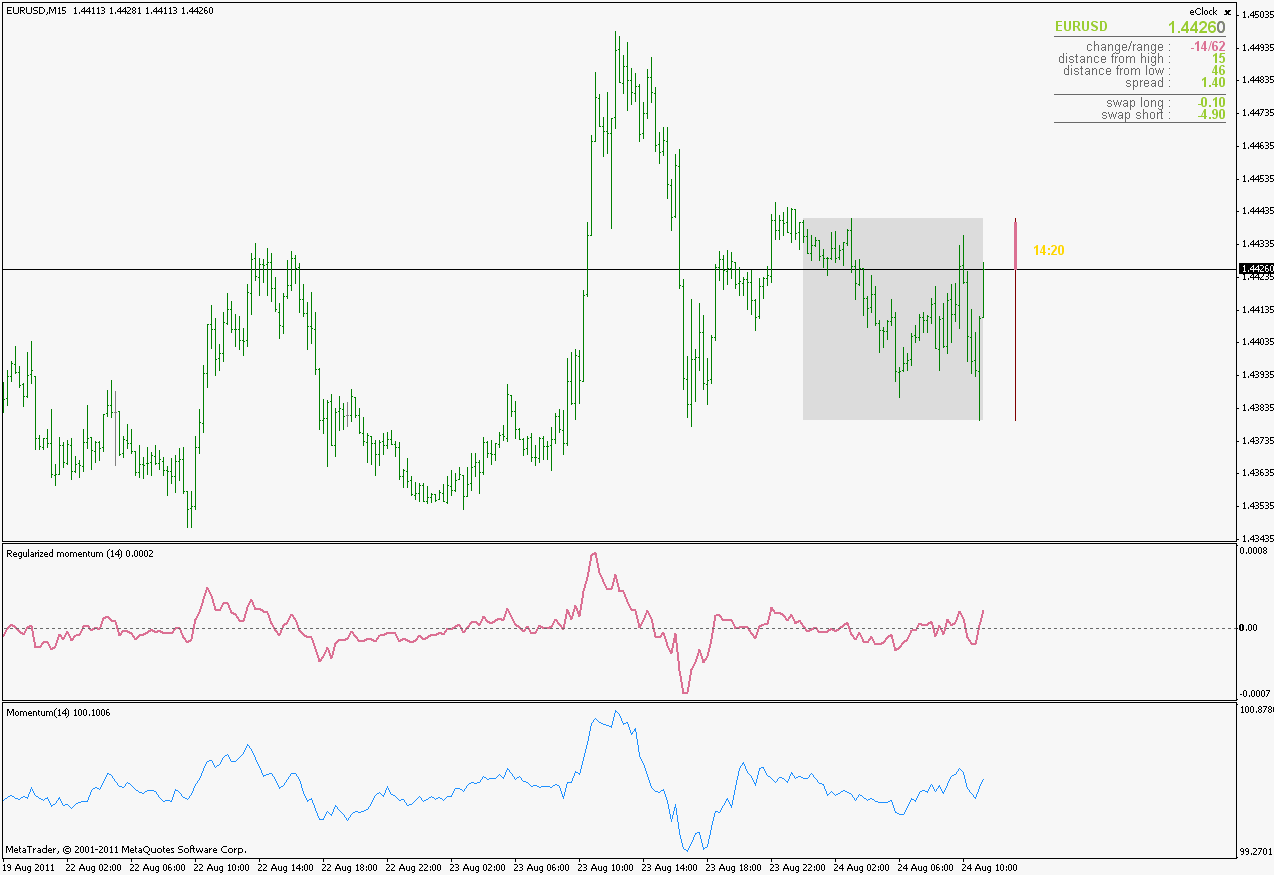The same thing with Lambda set to 5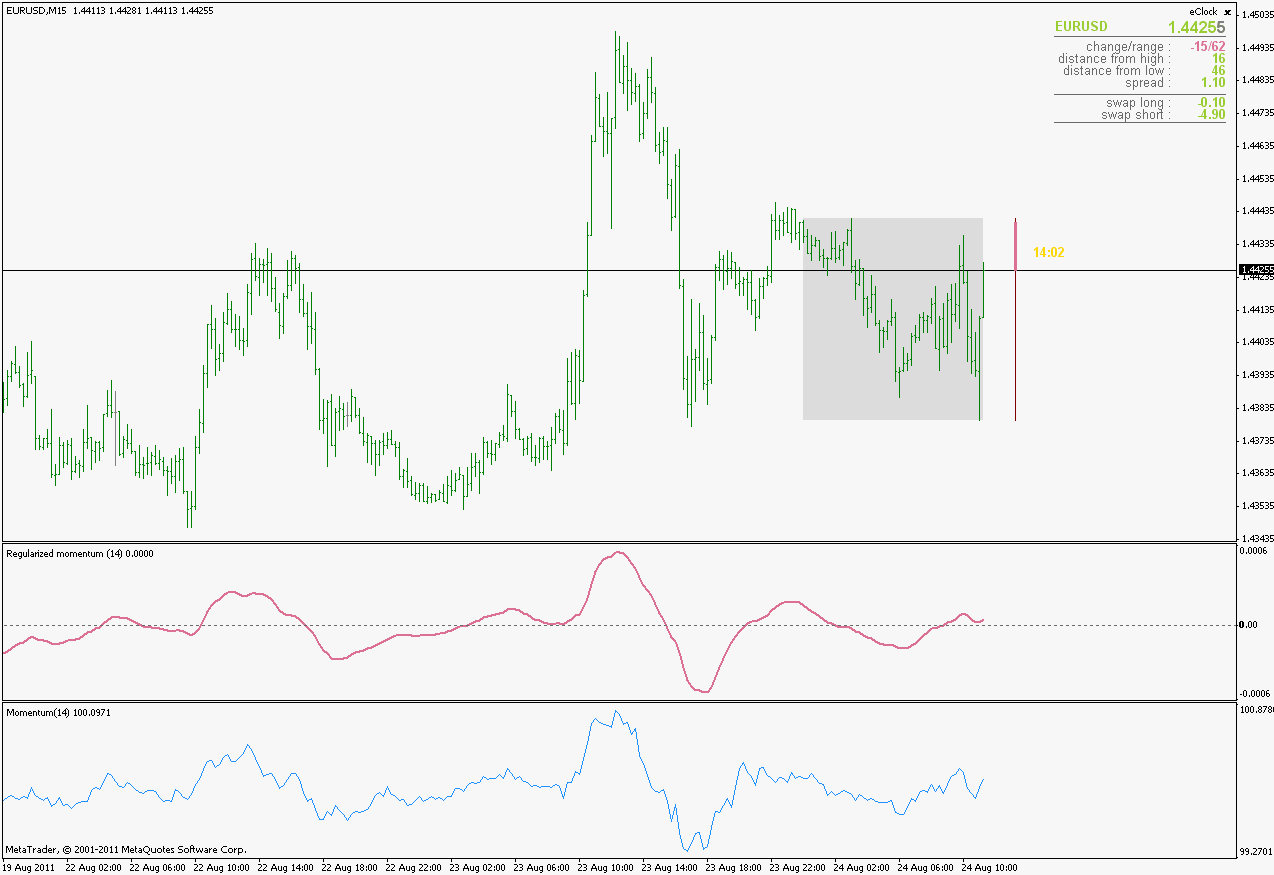And the same thing with Lambda set to 10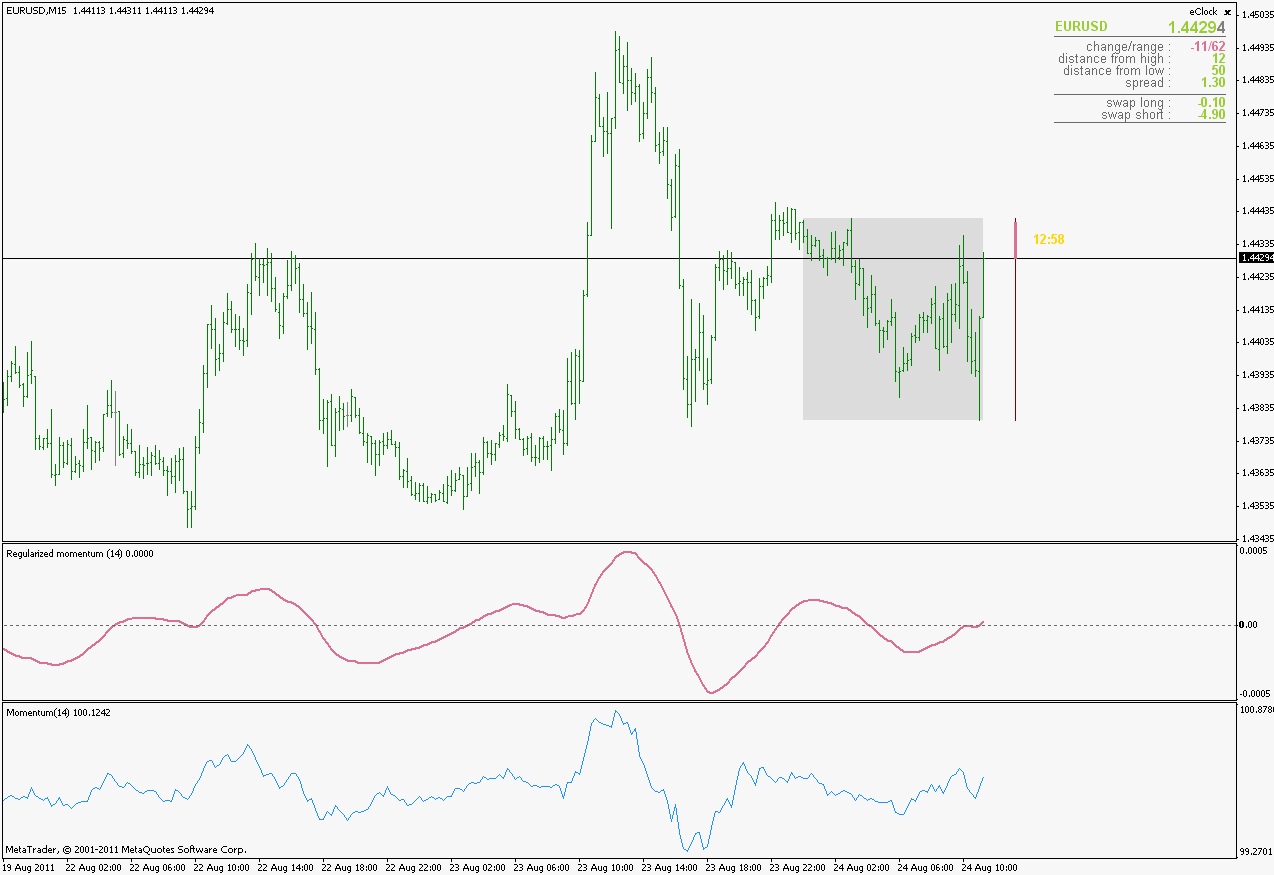It is obvious that, unlike the original momentum indicator, this one even has a "signaling capability" (zero line crosses). Besides of being much smoother and clearer to see what the momentum actually is, with signaling possibility (when not over smoothed) a lot of false signals is filtered out and thus it becomes a rather useful tool for trading

Files:
rm_1.gif  25 kb
rm_2.gif  24 kb
rm_3.gif  24 kb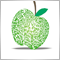106

Regularized Momentum

Hi mlaben ,

many thanks for all your fine Mt4 indicators.

I have the Metastock code : it is not same ?

Regularized Momentum

x:=Input("number of periods in moving average",1,500,21);

d:=Input("regularization constant",0,20,.5);

a:=2/(x+1);

f:=(PREV*(1+(2*d))+(a*(C-PREV))-(d*Ref(PREV,-1)))/(1+d);

(f-Ref(f,-1))/f

Regards.255627

sohocool

It is not different - they are exactly the sameThe only thing that differs is the Lambda parameter name: in metastock code they call it "regularization constant" and it is set to default 0.5 (as in the original Regularized moving average) while I use default value of 5.0 instead.

As of Lambda : Lambda parameter name was used by Chris Satchwel in his original article, so I did not feel any need to change its name, and as far as the default 0.5 for lambda : frankly I find the 0.5 way to sensitive for a zero cross based momentum - as I said, a bit more smoothing with minimal or no lag addition is preventing a lot of false signals in this indicator

regards527

Hi there

Looking for a leading indi: You will know that the Momentum indi is a suitable leading indi,- but it is to erratic most of the time. A solution is to use a smmoothed MA and use the slope to get the turning points at the high and loe of a trend.

I am looking for somebody who can alter a momentum indi in such a way that the input is not the bars values but the values of the MA which I add.

This indi could be posted here.

Any questions ( evtly for a fee) via email wolfgschilleratweb.de

Files:
smootrend.ex4  10 kb255627

...

If you are looking for a smooth momentum, you can download one from here : https://www.mql5.com/en/forum/180168 (Jurik velocity is in fact a smooth momentum) or one from few posts ago (regularized momentum, for example)

PS: if you are looking just for a momentum of T3 (the indicator you posted is a T3), then you can use this one : it is a momentum of T3. It allows different periods for T3 calculation and momentum calculation, so you can adjust almost everything in calculation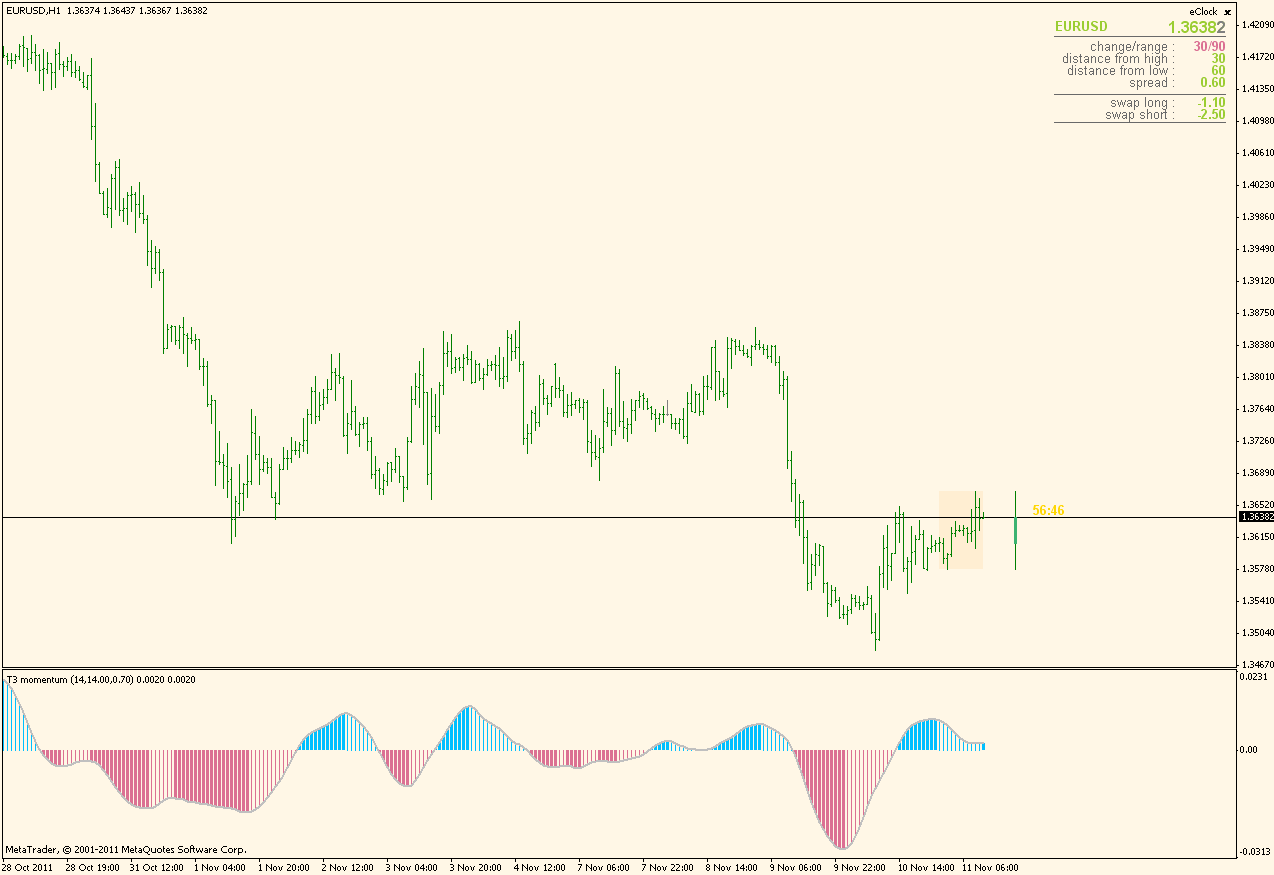PPS: this T3 allows fractional period for T3 (so it does not have to be an integer value)

regards

wolfsch:
Hi there

Looking for a leading indi: You will know that the Momentum indi is a suitable leading indi,- but it is to erratic most of the time. A solution is to use a smmoothed MA and use the slope to get the turning points at the high and loe of a trend.

I am looking for somebody who can alter a momentum indi in such a way that the input is not the bars values but the values of the MA which I add.

This indi could be posted here.

Any questions ( evtly for a fee) via email wolfgschilleratweb.de
Files: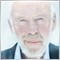694

Candlestick Momentum Index (CMI) indi ?

Hi there, I couldn't find an MQ4 implementation of the Blau CMI indicator so far. Formula is a double EMA smoothed candlestick momentum CM(i) = Close(i) – Open(i) as percentage value.

Another formula would be

CMI(i) = (EmaX(r) (EmaY(s) (CM(i))) * 100) / (EmaX(r) (EmaY(s) (Abs(CM(i)))) )

EmaXperiod = 32

EmaYperiod = 5

I would appreciate an implementation very much.255627

Blau Ergodic CMI

Thought that I posted it

Anyway, here it is : histogram part is the CMI, signal line is the ergodic addition (Blau always does like that : according to him 32,5,5 combination is Ergodic, all the rest is not)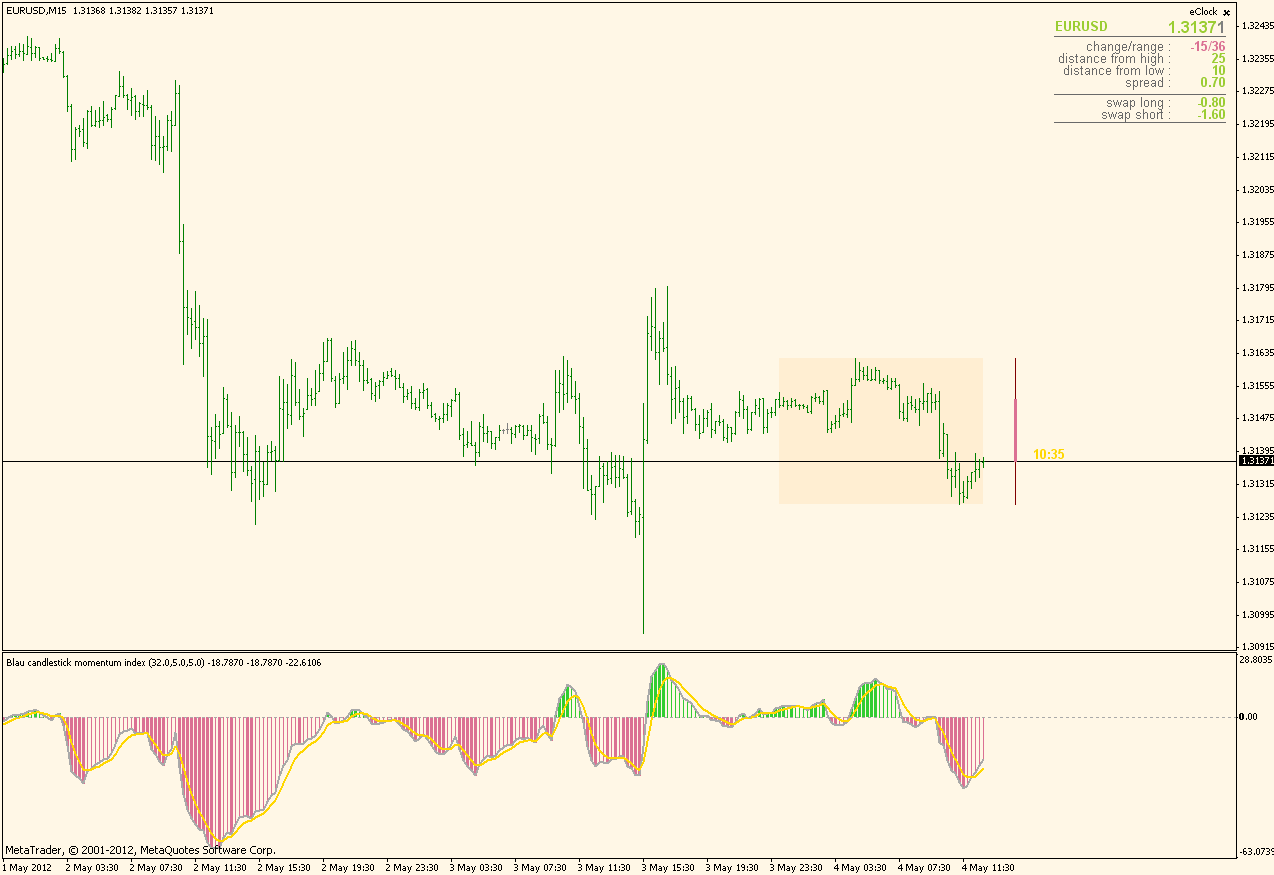Boxter:
Hi there, I couldn't find an MQ4 implementation of the Blau CMI indicator so far. Formula is a double EMA smoothed candlestick momentum CM(i) = Close(i) – Open(i) as percentage value.

Another formula would be

CMI(i) = (EmaX(r) (EmaY(s) (CM(i))) * 100) / (EmaX(r) (EmaY(s) (Abs(CM(i)))) )

EmaXperiod = 32

EmaYperiod = 5

I would appreciate an implementation very much.
Files:
blau_cmi.gif  25 kb255627

Multi pair version ...

Posted a multi pair variation (with some other variations too) of Blau CMI here : https://www.mql5.com/en/forum/178416/page13255627

Velocity ...

A very, very simple way to calculate velocity (what is sometimes called a Jurik velocity)

Just to remind that velocity is in fact a smoothed momentum which can be very helpfull since momentum tends to give some false signals due to its "rough" values calculated. Here is one comparison of the momentum (blue line) and velocity (red line).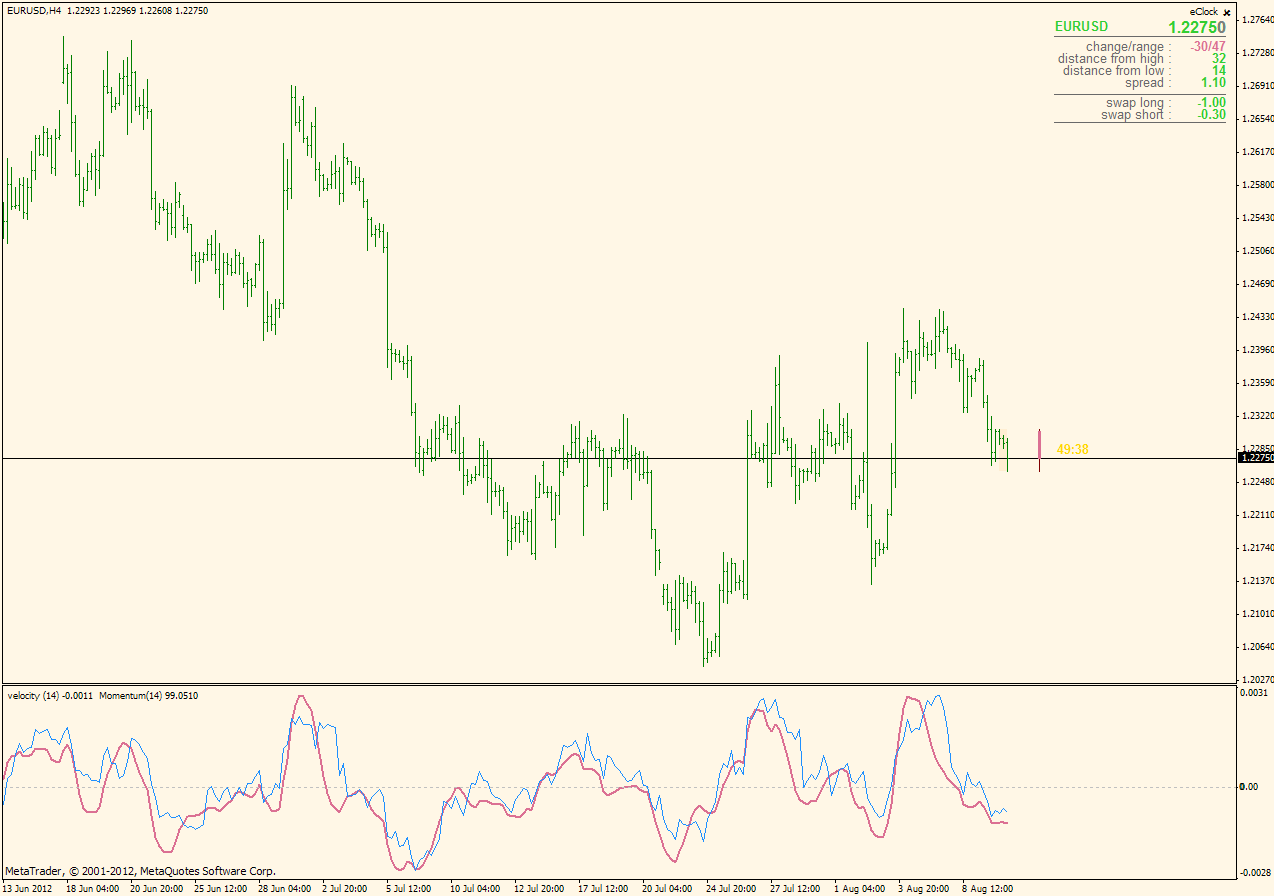PS: the way it is written, velocity can be easily calculated for any value, so in some sense, it covers the velocity and velocity on array in one single function

Files:
velocity.mq4  2 kb